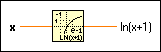# Natural Logarithm (Arg +1) Function

## LabVIEW 2018 Help

Edition Date: March 2018
Part Number: 371361R-01
View Product InfoLabVIEW 2016 HelpLabVIEW 2017 HelpLabVIEW 2018 HelpLabVIEW 2019 HelpLabVIEW 2020 Help

Owning Palette: Exponential Functions

Requires: Base Development System

Computes the natural logarithm of (x + 1).

When x is near 0, this function is more accurate than adding 1 to x then using the Natural Logarithm function. If x is equal to –1, the result is –. If x is not complex and is less than –1, the result is NaN. The connector pane displays the default data types for this polymorphic function.

Examplex can be a scalar number, array or cluster of numbers, array of clusters of numbers, and so on.ln(x+1) is of the same numeric representation as x. When x is of the form x = a + bi, that is, when x is complex, the following equation defines ln(x+1): ln(x+1) = ln(|x + 1|) + i arctan2(b, a + 1)

## Example

Refer to the Exponential VI in the labview\examples\Mathematics\Elementary & Special Functions\Exponential Functions directory for an example of using the Natural Logarithm (Arg +1) function.